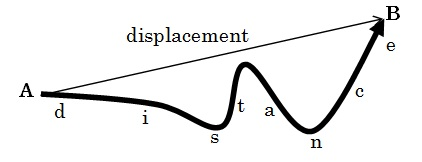# How to Solve Motion Problems Using Equations of Motion

To solve motion problems using equations of motion (under constant acceleration), one uses the four suvat” equations. We will look at how these equations are derived, and how they can be used to solve simple motion problems of objects traveling along straight lines.

## Difference Between Distance and Displacement

Distance is the total length of the path traveled by an object. This is a scalar quantity. Displacement ($s$) is the shortest distance between the object’s starting point and the final point. It is a vector quantity, and the direction of the vector is the direction of a straight line drawn from starting point to the final point.Using displacement and distance, we can define the following quantities:

Average speed is the total distance traveled per unit time. This is also a scalar. Unit: m s-1.$\mathrm{average\: speed=\frac{total\: distance\: traveled}{time\: taken}}$

Average velocity ($v$) is the displacement divided by the time taken. The direction of velocity is the direction of the displacement. Velocity is a vector and its unit: m s-1.$\mathrm{average\: velocity=\frac{displacement}{time\: taken}}$

Instantaneous velocity is the velocity of an object at a specific point in time. This doesn’t take the entire journey into account, but only the speed and direction of the object at the particular time (e.g. the reading on a car’s speedometer gives the speed at a specific time). Mathematically, this is defined using differentiation as:$v=\frac{\mathrm{d}s}{\mathrm{d}t}$

Example

A car is travelling at a constant speed of 20 m s-1. How long does it take to travel a distance of 50 m?

We have$time=\frac{speed}{distance}\Rightarrow time=\frac{20}{50}=0.4\; \mathrm{s}$.

## How to Find Acceleration

Acceleration ($a$) is the rate of change of velocity. It is given by$\mathrm{chnge\: in\: velocity=\frac{displacement}{time\: taken}}$

If the velocity of an object changes, we often use$u$ to denote the initial velocity and$v$ to denote final velocity. If this velocity change from  to  occurs during a time$t$, we can write$a=\frac{v-u}{t}$

If you get a negative value for acceleration, then the body is decelerating or slowing down. Acceleration is a vector and has units m s-2.

Example

An object, traveling at 6 m s-1, is subjected to a constant deceleration of 0.8 m s-2. Find the speed of the object after 2.5 s.

Since the object is decelerating, it’s acceleration should be taken to have a negative value. Then, we have$u=6\:\mathrm{m\: s^{-1}},\: a=-0.8\:\mathrm{m\: s^{-2},\:}t=2.5\:\mathrm{s}.\: v=\mathrm{?}$.$v=u+at\Rightarrow v=6-\left(0.8\times2.5\right)=6-2=4\mathrm{\: m\: s^{-1}.}$.

## Equations of Motion with Constant Acceleration

In our subsequent calculations, we will consider objects experiencing a constant acceleration. To do these calculations, we will use the following symbols:$u=$ the object’s initial velocity$v=$ the object’s final velocity$s=$ the object’s displacement$a=$ the object’s acceleration$t=$ time taken

We can derive four equations of motion for objects experiencing constant acceleration. These are sometimes called suvat equations, because of the symbols that we use. I will derive these four equations below.

Starting with$a=\frac{v-u}{t}$ we rearrange this equation to get:$v=u+at$

For an object with constant acceleration, the average velocity can be given by$\frac{u+v}{2}$. Since displacement = average velocity × time, we then have$s=\left(\frac{u+v}{2}\right)t$

Substituting$v=u+at$ in this equation, we get,$s=\left(\frac{u+u+at}{2}\right)t$

Simplifying this expression yields:$s=ut+\frac{1}{2}at^2$

To obtain the fourth equation, we square$v=u+at$:$v^2=u^2+2uat+a^2t^2$$\Rightarrow v^{2}=u^{2}+2a\left(ut+\frac{1}{2}at^{2}\right)$$v^2=u^2+2as$

## How to Solve Motion Problems Using Equations of Motion

To solve motion problems using equations of motion, define a direction to be positive. Then, all vector quantities pointing along this direction are taken as positive and the vector quantities pointing in the opposite direction are taken to be negative.

Example

A car increases its velocity from 20 m s-1 to 30 m s-1 while traveling a distance of 100 m. Find the acceleration.

We have$u=20,\: v=30,\: s=100,\: a=?$$v^{2}=u^{2}+2as\Rightarrow a=\frac{v^{2}-u^{2}}{2s}=\frac{400-900}{200}=2.5\:\mathrm{m\: s^{-2}}$.

Example

After applying emergency breaks, a train traveling at 100 km h-1 decelerates at a constant rate and comes to a rest in 18.5 s. Find how far the train travels, before it comes to rest.

The time is given in s, but the speed is given in km h-1. So, first we will convert 100 km h-1 to m s-1$100\: km\: h^{-1}=\frac{100000\: m}{3600\: s}=27.8\: m\: s^{-1}$.

Then, we have$u=28.7,\: v=0,\: t=18.5,\: s=?$$s=\frac{1}{2}(u+v)t\Rightarrow s=\frac{1}{2}\times(28.7+0)\times18.5=265\:\mathrm{m}$

Same techniques are used to do calculations on objects falling at free fall. Here, the acceleration due to gravity is constant.

Example

An object is thrown object vertically upwards at a speed of 4.0 m s-1 from the ground level. The acceleration due to the Earth’s gravity is 9.81 m s-2. Find how long it takes for the object to land back on the ground.

Taking upwards direction to be positive, the initial velocity$u=4.0$ m s-1. Acceleration is towards thee ground so$a=-9.81$ m s-2. When the object falls, it has moved back to the same level, so. So$s=0$ m.

We use the equation$s=ut+\frac{1}{2}at^2$. Then,$0=4t-\frac{1}{2}\times9.81\times t^{2}\Rightarrow0=4t-4.91t^{2}$. Then,$0=t(4-4.91)$. Then$t=$ 0 s or 0.82 s.

The “0 s” answer refers to the fact that, at the beginning (t=0 s), the object was thrown from the ground level. Here, the object’s displacement is 0. The displacement becomes 0 again when the object comes back to the ground. Then, the displacement is again 0 m. This happens 0.82 s after it was thrown up.

## How to Find the Velocity of a Falling Object